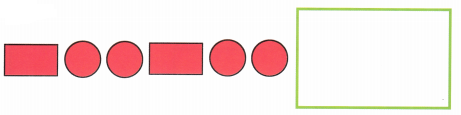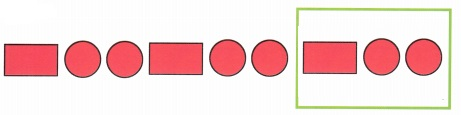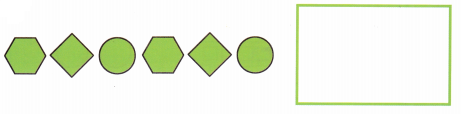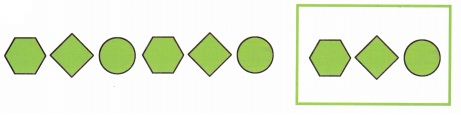# Math in Focus Kindergarten Chapter 7 Answer Key Solid and Flat Shapes

Go through the Math in Focus Grade K Workbook Answer Key Chapter 7 Solid and Flat Shapes to finish your assignments.

## Math in Focus Kindergarten Chapter 7 Answer Key Solid and Flat Shapes

Lesson 1 Solid Shapes

Which shape is it? Color.

Question 1.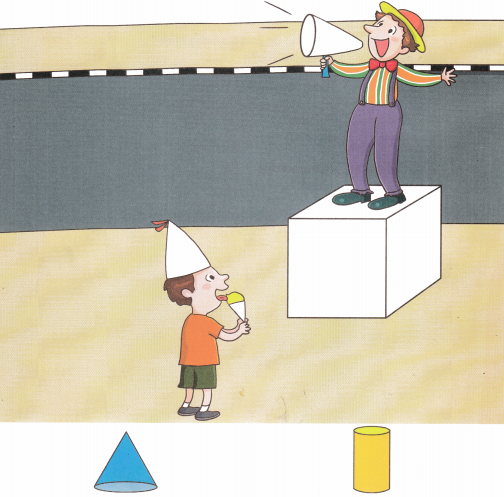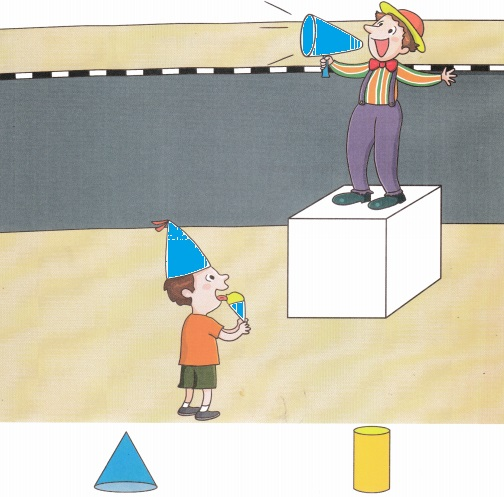Explanation:
Cone Shape,
Colored Icecream, Cap, Announcement Speaker.

Question 2.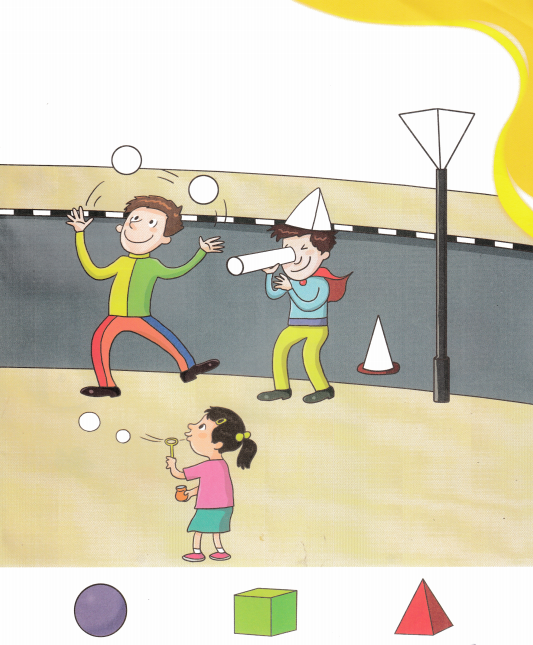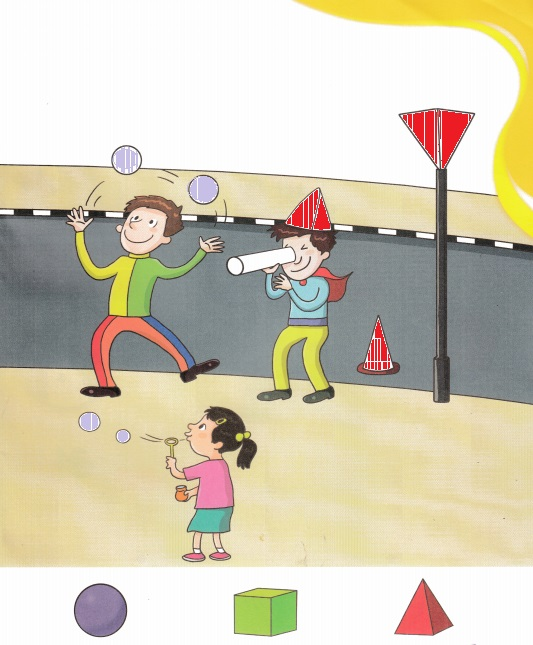Explanation:
Circle shape – Balls, Bubbles,
Cone shape – Caps, Lamp.

Lesson 2 Flat Shapes in Solid Shapes

Match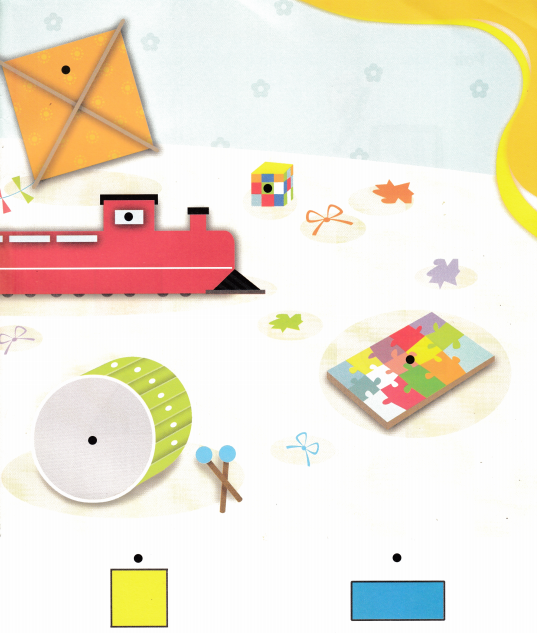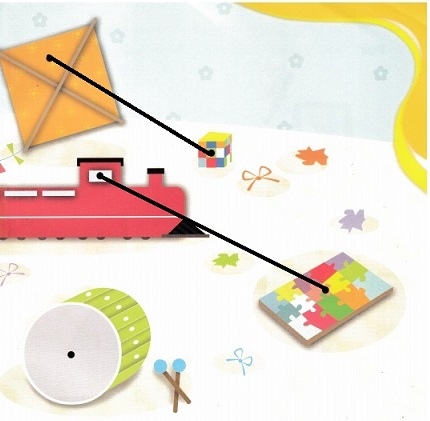Explanation:
Matched with the given solid shapes,
Cube- Square,
Rectangle – Game Puzzel.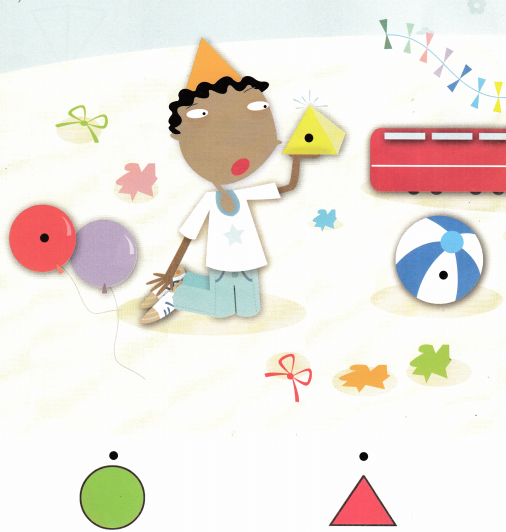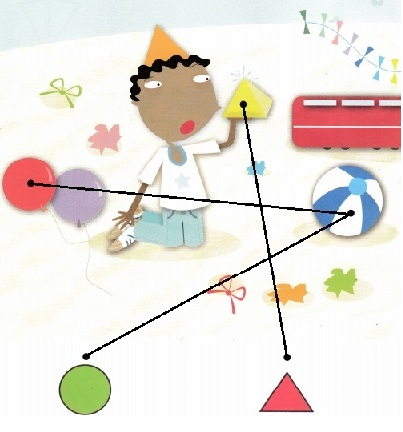Explanation:
Matched with the given solid shape
Round-Circle- Balloons, Ball,
Triangle –  Prism.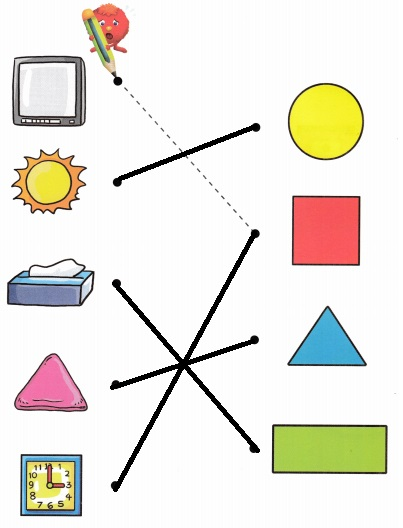Pair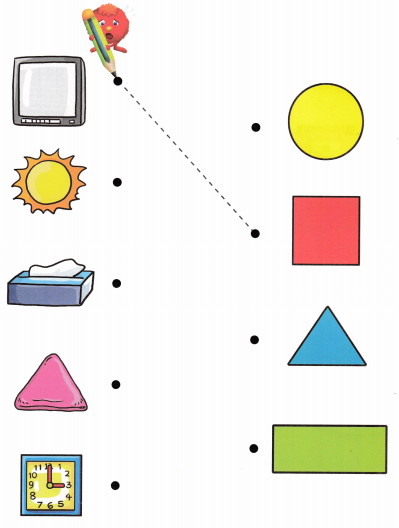Explanation:
Circle – Sun,
Rectangle – Tissue Box,
Triangle – Prism,
Sqaure – Clock.

Lesson 3 Flat Shapes

Draw

Question 1.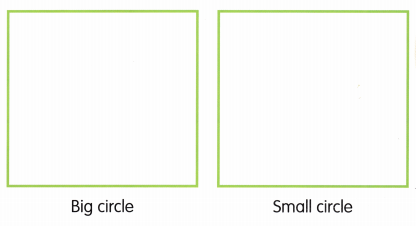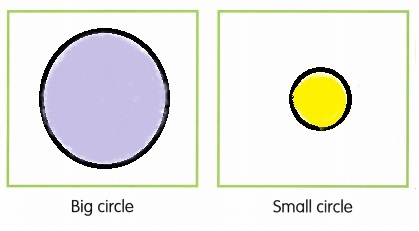Explanation:
Drawn Big Circle and Small Circle in the given space.

Question 2.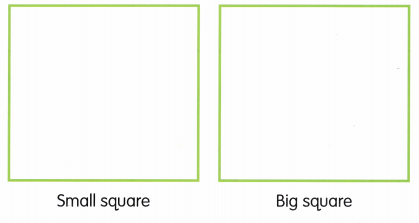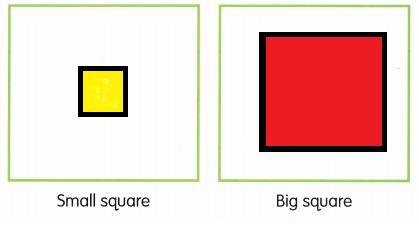Explanation:
Drawn Small Sqaure and Big Square in the given place.

Question 3.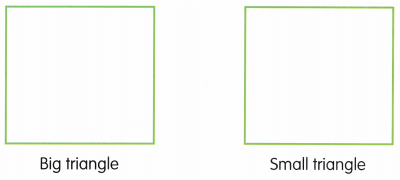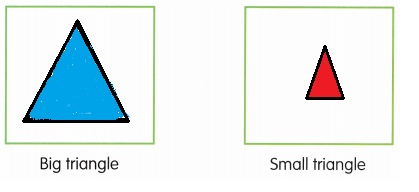Explanation:
Drawn Big Triangle and Small Triangle in the given space.

Question 4.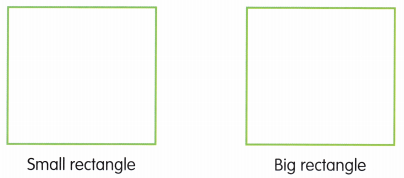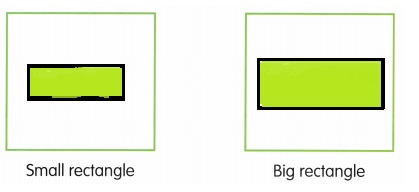Explanation:
Drawn Small Rectangle and Big Rectangle in the given space.

Question 5.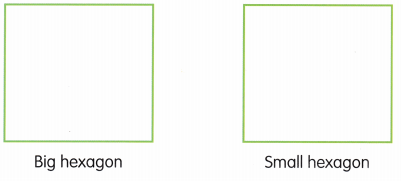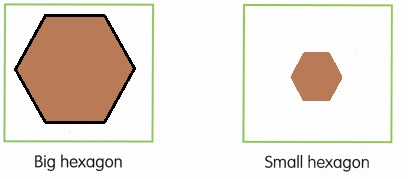Explanation:
Drawn Big Hexagon and Small Hexagon in the given space.

Lesson 4 Flat Shape Pictures

Color the squares red. Color the rectangles green. Color the circle yellow.
Color the triangle blue. Color the hexagon brown.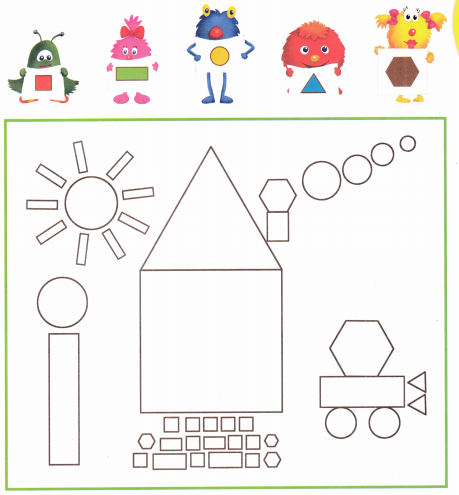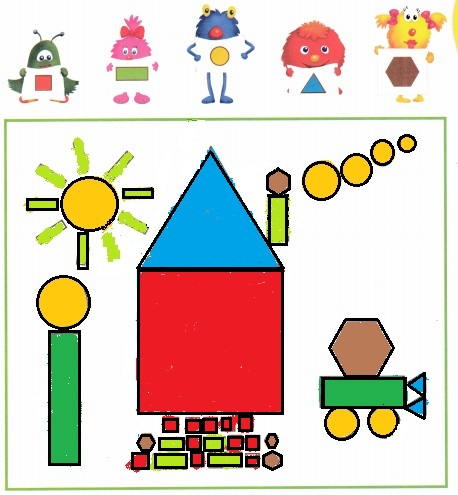Explanation:
Colored the squares with red. Colored the rectangles with green.
Colored the circles with yellow.
Colored the triangles with blue. Colored the hexagons with brown.

Lesson 5 Shape Patterns

Complete the pattern.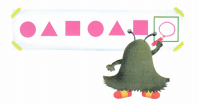Question 1.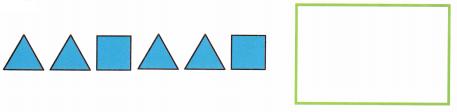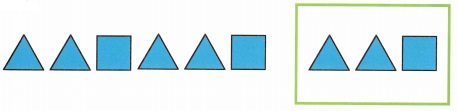Explanation:
Completed the given pattern in the given box.

Question 2.